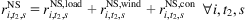(3.64)

${r}_{i,{t}_{2},s}^{\mathrm{NS}}={r}_{i,{t}_{2},s}^{\mathrm{NS},\mathrm{load}}+{r}_{i,{t}_{2},s}^{\mathrm{NS},\mathrm{wind}}+{r}_{i,{t}_{2},s}^{\mathrm{NS},\mathrm{con}}\forall i,{t}_{2},s$ (3.65)(3.65)

$0\le {r}_{i,{t}_{2},s}^{\mathrm{UP}}\le {R}_{i,{t}_{1}}^{\mathrm{UP}}\forall i,{t}_{2}\in {T}_{2}^{\mathrm{in}},{t}_{1},s$ (3.66)(3.66)

$0\le {r}_{i,{t}_{2},s}^{\mathrm{DN}}\le {R}_{i,{t}_{1}}^{\mathrm{DN}}\forall i,{t}_{2}\in {T}_{2}^{\mathrm{in}},{t}_{1},s$ (3.67)(3.67)

$0\le {r}_{i,{t}_{2},s}^{\mathrm{NS}}\le {R}_{i,{t}_{1}}^{\mathrm{NS}}\forall i,{t}_{2}\in {T}_{2}^{\mathrm{in}},{t}_{1},s$ (3.68)(3.68)

The power output of a ...

Get Optimization in Renewable Energy Systems now with the O’Reilly learning platform.

O’Reilly members experience books, live events, courses curated by job role, and more from O’Reilly and nearly 200 top publishers.